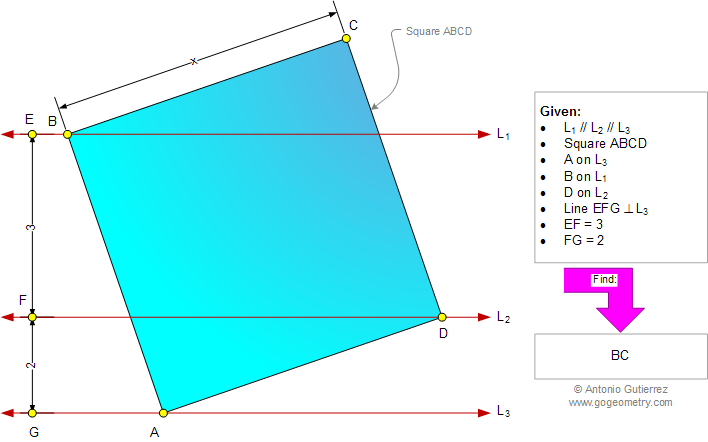# Geometry Problem 973: Three Parallel, Square, Vertices, Distance Between Two Parallel Lines, Side, Metric Relations. Level: School, College, Mathematics Education

< PREVIOUS PROBLEM  |  NEXT PROBLEM >

 Lines L1, L2, and L3 are parallel (Figure below) with line EFG perpendicular to L3. ABCD is a square with A, B, and D on, L3, L1, and L2, respectively. If EF = 3 and FG =2, find the side of the square.Home | Search | Geometry | Problems | All Problems | Open Problems | Visual Index | 10 Problems | Problems Art Gallery |  Art | 971-980 | Square | Triangles | Right Triangle | Parallel lines | Perpendicular lines | Metric Relations | by Antonio Gutierrez